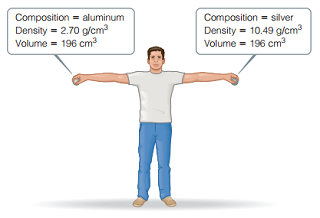# Problem: Consider the two spheres shown here , one made of silver and the other of aluminum. The spheres are dropped from a height of 2.1 m.What is the velocity of the aluminum sphere at the moment it hits the ground? (Assume that energy is conserved during the fall and that 100% of the sphere’s initial potential energy is converted to kinetic energy by the time impact occurs.)

###### FREE Expert Solution

Potential energy:

$\overline{)\mathbf{PE}\mathbf{=}\mathbf{mgh}}$

Kinetic energy:

$\overline{)\mathbf{KE}\mathbf{=}\frac{\mathbf{1}}{\mathbf{2}}{\mathbf{mv}}^{\mathbf{2}}}$

For aluminum:

energy is conserved during the fall and that 100% of the sphere’s initial potential energy is converted to kinetic energy

89% (311 ratings)###### Problem Details

Consider the two spheres shown here , one made of silver and the other of aluminum. The spheres are dropped from a height of 2.1 m.What is the velocity of the aluminum sphere at the moment it hits the ground? (Assume that energy is conserved during the fall and that 100% of the sphere’s initial potential energy is converted to kinetic energy by the time impact occurs.)